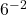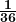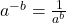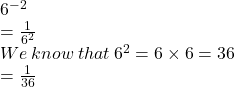## Rewrite the following without an exponent. 6^-2 Please answer ASAP!

Question

Rewrite the following without an exponent. 6^-2

in progress 0
5 months 2021-08-25T12:23:48+00:00 1 Answers 4 views 0

## Answers ( )

Rewritingwithout an exponent we getStep-by-step explanation:

We need to rewrite the following without an exponent.We need to solve the exponent to find the result.

We know the exponent rule:Now using this rule to solve the equation:So, Rewritingwithout an exponent we get